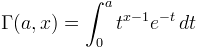Computes the upper incomplete Gamma function.

Namespace:  Meta.Numerics.Functions
Assembly:  Meta.Numerics (in Meta.Numerics.dll) Version: 4.1.4Syntax
```public static double Gamma(
double a,
double x
)```

#### Parameters

a
Type: SystemDouble
The shape parameter, which must be positive.
x
Type: SystemDouble
The argument, which must be non-negative.

#### Return Value

Type: Double
The value of Γ(a,x).Remarks

The incomplete Gamma function is defined by the same integrand as the Gamma function (Gamma(Double)), but the integral is not taken over the full positive real axis.Like the Γ function itself, this function gets large very quickly. For many purposes, you will prefer to use the regularized incomplete gamma functions LeftRegularizedGamma(Double, Double) and RightRegularizedGamma(Double, Double), which are accurately computed even in regions for which this function overflows.See Also